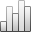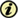Acta Stereologica

0351-580XMore Statisticssince 05 December 2013 :
View(s): 68 (0 ULiège)
Mohsen Shahinpoor

Error estimation in stereological determination of particle size distribution

ArticleAbstract

Stereological determination of particle size distribution normally amounts to solving a Volterra's integral equation of the first kind. Computationally speaking, this integral equation must be discretized and converted into a set of n algebraic equations in n unknowns. As the value of n increases the accuracy of estimation also increases. Here is proposed a technique of replacing the distribution density f(r) obtained from randomly intersecting planes with cumulative distribution density φ(r). Here φ(r) is the number of cross-sections with radii equal to or smaller than r per unit area. Similarly Φ(R) is defined as the number of spheres with radii equal to or smaller than R per unit volume. The integral equation is thus reduced to an Abel's type. Finally, the error involved in discrete approximation with large n is obtained and the best formula to minimize the error is derived.